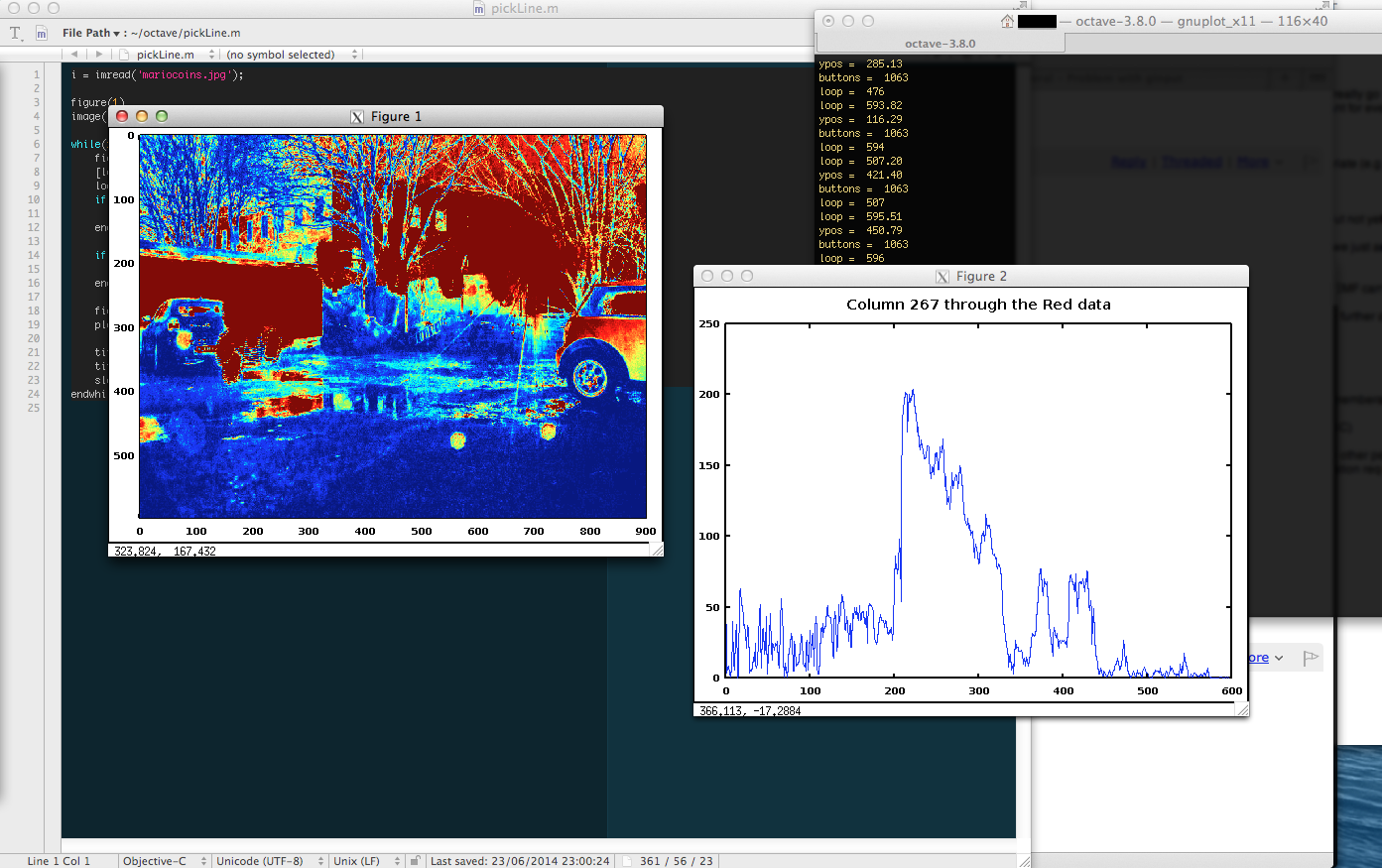Here’s a trick I used to present multi-dimensional data with the Octave mathematical programming language. In my particular application, I had a dataset that varies with respect to time, and wanted to examine the structure making up one of the data points at a particular time interval.I used the `ginput` command to detect clicks on the main dataset and then display the sub-structure on a second plot panel. By way of making a tangible example, here’s some code to basically the same thing which selects a column in an image.

Here’s the code:

``````#Read an image file and display the first channel in an image plot
figure(1)
image(i(:,:,1));

while(1)
figure(1)  #Select the figure containing the image
[loop, ypos, buttons] = ginput(1) #get the mouse position
loop = round(loop)
if(loop<1)
loop=1
endif

if(loop > columns(i))
loop = columns(i)
endif

figure(2) #Select the figure containing the graph and plot to it.
plot(i(:,loop,1))

titleText = sprintf('Column %i through the Red data', loop);
title(titleText, 'fontsize', 14)
sleep(0.1) #Don't kill your performance
endwhile
``````

If you’re on a Mac, you need to use `setenv ("GNUTERM", "x11") ` to make sure that your grphics terminal interacts with your mouse (the default AquaTerm doesn’t). On Windows and Mac, make sure that your graphics toolkit is gnuplot.